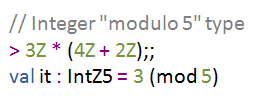TP

# F# Math - Numerical computing and F# PowerPackThis article is the first article of a series where I'll explain some of the F# features that are useful for numeric computing as well as some functionality from the F# PowerPack library. Most of the content was originally written for the Numerical Computing in F# chapter on MSDN (that I announced earlier), but then we decided to focus on using F# with third party libraries that provide more efficient implementation and richer set of standard numeric functionality that's needed when implementing machine learning and probabilistic algorithms or performing statistical analysis. If you're interested in these topics, then the last section (below) gives links to the important MSDN articles.

However, F# PowerPack still contains some useful functionality. It includes two additional numeric types and an implementation of matrix that integrates nicely with F#. The series also demonstrates how to use features of the F# language (and core libraries) to write numeric code elegantly. In particular, we'll use the following aspects:

• Overloaded operators. Any type can provide overloaded version of standard numeric operators such as +, -, * and / as well as other non-standard operators (such as .*). As a result, libraries can implement their own numeric types which are indistinguishable from built-in types such as int.
• Numeric literals. F# math libraries can enable using new numeric literals in the code. For example, you can write a BigRational value representing one third as 1N/3N. The N suffix used in the notation is not hardcoded in the F# language and we'll see how to define similar numeric type.
• Static constraints. F# supports static member constraints, which can be used for writing functions that work with any numeric type. For example, the List.sum function uses this feature. It can sum elements of any list containing numbers.

These are just a few of the F# language features that are useful when writing numeric code, but there are many others. The usual F# development style using interactive tools, type safety that prevents common errors, units of measure as well the expressivity of F# make it a great tool for writing numeric code. For more information, take a look at the MSDN overview article Writing Succinct and Correct Numerical Computations with F#.

## Numerical computing and F# PowerPack

If you're looking for information about other PowerPack components, then Daniel Mohl (@@dmohl) wrote a series that covers numerical types and modules (Part 1), asynchronous extensions (Part 2), additional collection types (Part 3), as well as lexing, parsing and SI units (Part 4).

In this article series, we look at most of the numerical computing features provided by the F# PowerPack library. The following list shows the upcoming articles of the series:

• ### F# Math (I.) - Numeric types in PowerPack

The PowerPack library provides two additional numeric types. The type BigRational type represents arbitrary precision rational numbers and complex represents complex numbers. This article briefly demonstrates both of the types. The version for .NET 2.0 also provides arbitrary size integers (which are available for .NET 4 in the System.Numerics.dll assembly).

• ### F# Math (II.) - Using matrices for graph algorithms

The matrix type can be used for working with matrices that store floating point numbers. It supports many of the common operations such as matrix multiplication, point-wise addition and others. There is also a generic variant of the type named Matrix<'T>, which can be used for working with matrices that contain values of type int or decimal. This article demonstrates how to use the matrix type to represent a graph using an adjacency matrix and how to calculate reachability in the graph.

• ### F# Math (III.) - Defining custom numeric types

This step-by-step tutorial shows how to define a numeric type that can be written using a custom numeric literal and can be used with standard operators. The tutorial also uses GlobalAssociations module from F# PowerPack to allow using the custom type as an element of F# Matrix<'T> type.

• ### F# Math (IV.) - Writing generic code  (Not available yet)

The article discusses two options for writing generic code that works with an any numeric type (such as float and complex). The first approach is based on F# static member constraints and the second is based on the GlobalAssociations module from F# PowerPack. In this article, we compare both options and discuss which approach is preferable in what cases.

## Numerical Computing in F# (MSDN)

As already mentioned, F# PowerPack provides only basic implementation of matrices, which is not suitable for tasks that require efficient matrix multiplication. The library also does not implement any advanced operations such matrix decomposition or any support for working with probability distributions or statistical analysis.

If you're interested in writing highly-efficient numeric code in F#, then it is recommended to use a third-party library (such as open-source Math.NET Numerics) that provide more efficient implementation and wider range of features. The MSDN section Introduction to Numerical Computing in F# provides more information including a review of existing libraries. It also includes examples that demonstrate the following three options:

• ### Tutorial: Using Math.NET Numerics in F#

Math.NET Numerics aims to be the standard open source math library for .NET Framework. It is released under the MIT license and so it can be used in both commercial and non-commercial projects, as well as modified and redistributed. This tutorial gives an introduction on how to use the various components of this library.

• ### Tutorial: Using Microsoft Sho in F#

Microsoft Sho is a numerical computing platform with support for linear algebra, optimization, and visualization. This article gives an introduction to Sho and shows how to call its .NET libraries from F#.

• ### Tutorial: Using the F# PowerPack and the F# MathProvider

The F# PowerPack provides types for vectors and matrices, and the F# MathProvider supports linear algebra operations on these types. This article presents an introduction to the two libraries.